# Jacobi brackets

(diff) ← Older revision | Latest revision (diff) | Newer revision → (diff)

Mayer brackets

The differential expression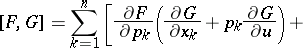(1)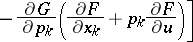in the functions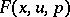and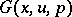of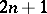independent variablesand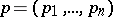.

The main properties are:

1)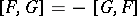;

2)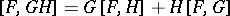;

3) if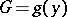,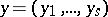and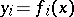, then;

4)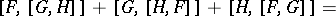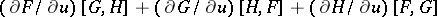.

The last property is called the Jacobi identity (see , ).

The expression (1) is sometimes written in the form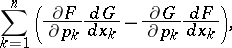where the symbolic notation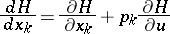(2)

is used. Ifandare regarded as functions of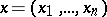, and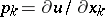,, then (2) gets the meaning of the total derivative with respect to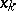.

Ifandare independent of, then their Jacobi brackets (1) are Poisson brackets.

How to Cite This Entry:
Jacobi brackets. Encyclopedia of Mathematics. URL: http://encyclopediaofmath.org/index.php?title=Jacobi_brackets&oldid=12643
This article was adapted from an original article by A.P. Soldatov (originator), which appeared in Encyclopedia of Mathematics - ISBN 1402006098. See original article